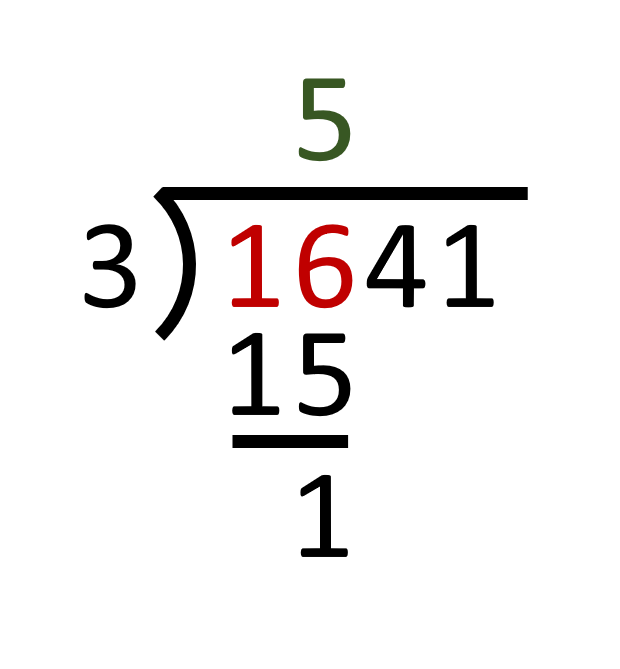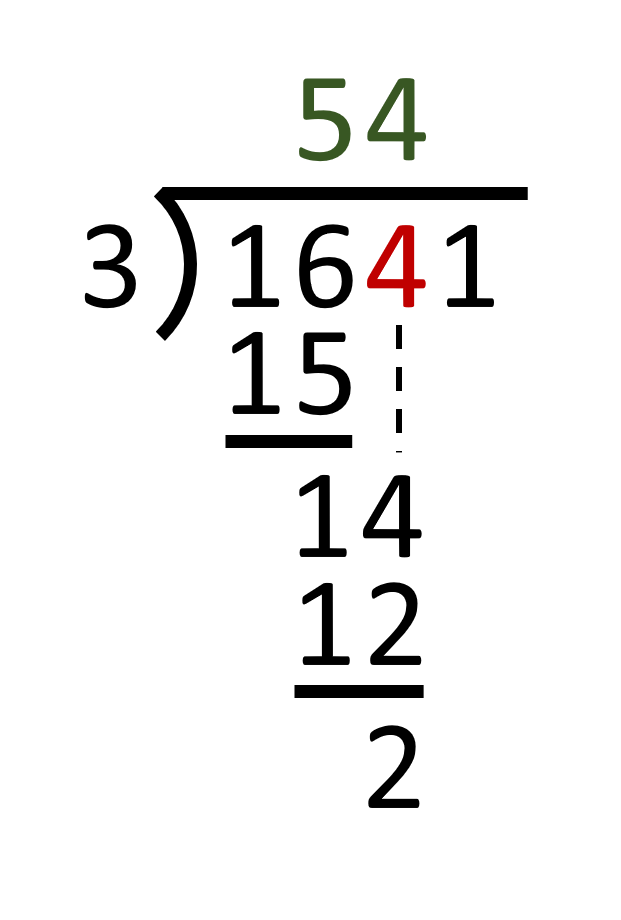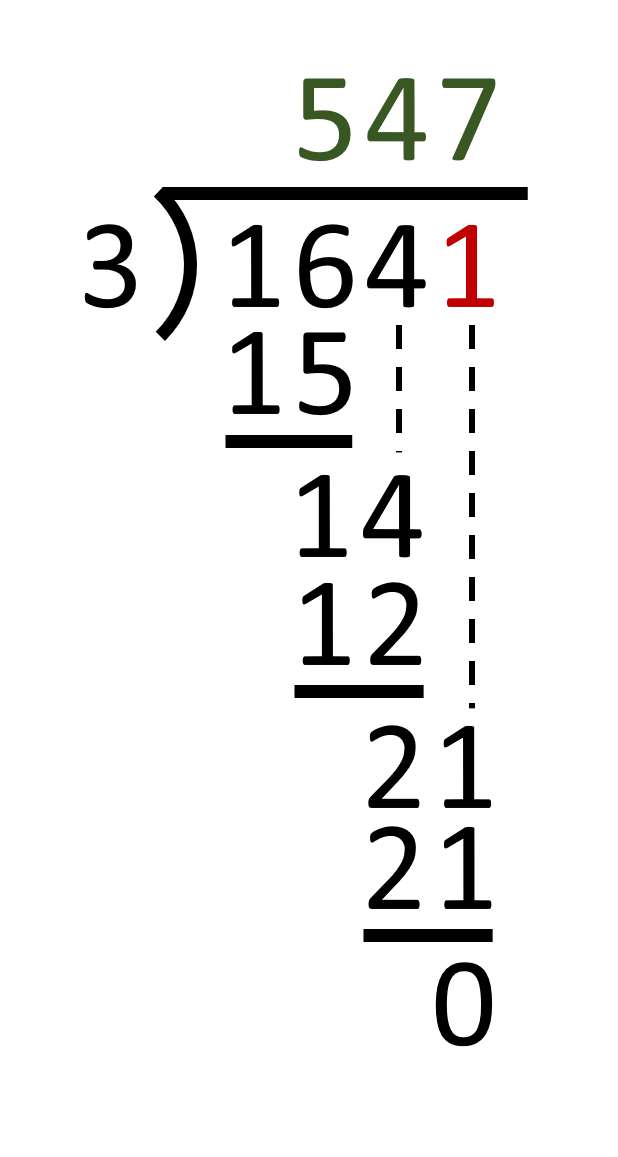## Try the long division calculator

The Long division calculator shows the complete work for dividing the dividend by the divisor producing the quotient. A long division is a method for dividing multidigit numbers by hand. It breaks down the division into a series of easier steps. The long division calculator shows the complete series of steps of the this method for dividing multidigit numbers.

# How to do long division

A long division is a method for dividing multidigit numbers by hand. It breaks down the division into a series of easier steps. One number, called the dividend, is divided by another number, called the divisor, producing the result called the quotient.

We will use an example to show you how to do a long division. We will divide 1641 by 3.The long division starts by dividing the left-most digit of the dividend by the divisor. The left-most digit is 1. If we divide 1 by 3, the quotient, which has to be rounded down to an integer, would be 0. Therefore, in this case, we take the first and second left-most digit of the dividend as one number: 16. If we divide 16 by 3, the quotient (rounded down to an integer) is 5. This becomes the first digit of the result. We place the number 5 above the second left-most digit of the dividend (we skip the first left-most digit, because the result of 1 divided by 3 was 0). The product of 3 times 5 is 15, which is placed below the 16. Next the 15 under is subtracted from the 16 to get the remainder, 1. This remainder carries forward when the process is repeated on the following digit of the dividend (notated as 'bringing down' the next digit to the remainder).Now we bring down the next digit (4) and place it next to the remainder (1), which gives us the number 14. If we divide 14 by 3, the quotient is 4. This becomes the next digit of the result. 3 times 4 is 12, which leaves us a remainder of 2.Finally we bring down the last digit of the dividend (1). This gives us 21 to be divided by 3 resulting in 7 to be the last digit of the result. As 3 time 7 is 21, the remainder is 0. When all digits have been processed and no remainder is left, the process is complete. The result of this long divison is 547.